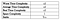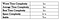# Asymptotic Analysis and Comparison of Sorting Algorithms

What is a Sorting Algorithm? A Sorting Algorithm is used to rearrange a given array or list of elements, usually according to a comparison operator on the elements. The comparison operator is used to decide the new order of elements in the respective data structure.

The efficiency of an algorithm is mainly defined by two factors, space and time. An efficient algorithm is one that takes less time and less space, but this is not possible simultaneously all of the time. There is often a trade-off between time and space. If you want to reduce the time, then space might increase. Similarly, if you want to reduce the space, then the time may increase. You may have to compromise and evaluate the tradeoffs.

# Big O Notation

Big O notation describes how many steps an algorithm takes based on the number of elements that are acted upon. For example, constant notation shows an algorithm will always take the same number of steps regardless of how much data is passed in. Logarithmic notation shows the number of operations increases by one each time the data is doubled. Quadratic notation illustrates that if the input array has 1 element it will do 1 operation, if it has 10 elements it will do 100 operations, the operations increase at an exponential rate every time the input data increases by one.

The illustrations below show the comparisons and details of common big O notations.

# Space Complexity

Space complexity is equal to the sum of two components:

1. A fixed amount of space that is required to store certain data such as variables, constants, program size, etc. and is not dependent on the size of the problem
2. A variable amount of space that is required by variables and is totally dependent on the size of the problem (i.e. recursion stack space and dynamic memory allocation)

Space complexity is usually represented with big O notation, which we know considers the worst-case scenario. What you create takes up space, and we need to be prepared for the worst!

# Time Complexity

Like space complexity, time complexity is also affected by factors such as the operating system and hardware, that is completely independent of the algorithm itself.

Time complexity is commonly estimated by counting the number of elementary operations performed by the algorithm, supposing that each elementary operation takes a fixed amount of time to perform. Therefore, the amount of time taken and the number of elementary operations performed by the algorithm are assumed to differ by at most a constant factor, and the algorithm that performs a task in the smallest number of operations is considered the most efficient one.

As with space complexity, time complexity is also usually represented with big O notation, considering the worst-case scenario. What you create takes up time, and we need to be prepared for the worst!

# Bubble Sort*See information on Stability at the end of the blog

# Insertion Sort

It works by assuming the first element in the array is already sorted. It then selects the next element and compares this to all elements in the array. Every element that is greater than the selected element is shifted, and the selected element is inserted. This process is repeated until the array is sorted.

# Quick Sort

In quick sort, we find a pivot point in the array to compare all other elements in the array. A pivot can be selected in different ways, as the first, last, random, or median element. If an element is smaller than the pivot, it is moved before the pivot. If an element is greater than the pivot, it is moved after the pivot. This is done simultaneously from both ends of the array, with a left pointer (first element) and right pointer (last element), incrementing toward each other and swapping values should they be on the wrong side. Once this is complete, the process continues recursively on the sub arrays, created by dividing the array in half, or partitioning, until the entire array is sorted.

# Merge Sort

In merge sort we divide the array into n arrays until each of the arrays contain only one element. The smaller arrays are merged in order to produce a new array. This is repeated until there is only one array remaining, the original array in its sorted state.

# Heap Sort

Basically, a heap is a binary tree with some added rules. The added rules are that, first, the binary tree must be complete. This means that you have to fill all the nodes at one level before adding another one. Secondly, there must be a defined parent/child relationship with the element values of the heap. In a min heap, the value of the parent must be smaller than its children. In a max heap, the value of the parent must be greater than its children.

In heap sort, we build a max heap that makes sure the highest value element is at the top. That top element is then swapped with the last element of the heap. The top element is removed and stored on a sorted array. This process is repeated until only one element remains in the heap.

One important point is that heaps are not natively supported in JavaScript, therefore we implement heaps using arrays. Although heap sort is complex, its space complexity is excellent.

# Radix Sort

Radix sort is the answer. Radix sort is a non-comparison based sorting algorithm. The radix sort algorithm distributes integers into buckets based on a number’s significant digit or value (the radix) to avoid the comparison.

The idea of Radix Sort is to do digit by digit sort starting from least significant digit to most significant digit. In other words, the ones place digits are sorted in into buckets first, then the tens place, then the hundreds place, and so on. Radix sort uses another sorting method called counting sort as a subroutine to complete its sort.*n is the number of elements and k is the number of bits of memory required to represent the largest element in the array

# A Note on Stability

Sorting algorithms are not all created equal. Each have strengths and drawbacks, and many of which you can use to solve the same problem. Therefore, make sure you do your research and choose wisely! Happy Coding!

# References

## More from Mary Rachael Koenke

Software Engineer, NYC